# Search ResultsCollection Resources(907)

View
Selected filters:
• Algebra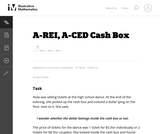Conditions of Use:
Remix and Share
Rating

The given solutions for this task involve the creation and solving of ...

The given solutions for this task involve the creation and solving of a system of two equations and two unknowns, with the caveat that the context of the problem implies that we are interested only in non-negative integer solutions. Indeed, in the first solution, we must also restrict our attention to the case that one of the variables is further even.

Subject:
Mathematics
Algebra
Material Type:
Activity/Lab
Provider:
Illustrative Mathematics
Provider Set:
Illustrative Mathematics
Author:
Illustrative Mathematics
Date created
1-جمادي الأولى-1434Conditions of Use:
Remix and Share
Rating

This task examines the ways in which the plane can be covered ...

This task examines the ways in which the plane can be covered by regular polygons in a very strict arrangement called a regular tessellation. These tessellations are studied here using algebra, which enters the picture via the formula for the measure of the interior angles of a regular polygon (which should therefore be introduced or reviewed before beginning the task). The goal of the task is to use algebra in order to understand which tessellations of the plane with regular polygons are possible.

Subject:
Mathematics
Algebra
Geometry
Material Type:
Activity/Lab
Provider:
Illustrative Mathematics
Provider Set:
Illustrative Mathematics
Author:
Illustrative Mathematics
Date created
3-جمادي الأولى-1434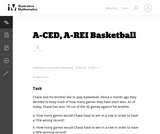Conditions of Use:
Remix and Share
Rating

This task provides a simple but interesting and realistic context in which ...

This task provides a simple but interesting and realistic context in which students are led to set up a rational equation (and a rational inequality) in one variable, and then solve that equation/inequality for an unknown variable.

Subject:
Mathematics
Algebra
Material Type:
Activity/Lab
Provider:
Illustrative Mathematics
Provider Set:
Illustrative Mathematics
Author:
Illustrative Mathematics
Date created
1-جمادي الأولى-1434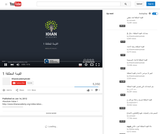Conditions of Use:
Remix and Share
Rating

This 3-minute video lesson introduces the concept of abolute value.

Subject:
Algebra
Material Type:
Lecture
Provider:
Provider Set:
Author:
Khan, Salman
Date created
25-ذو القعدة-1433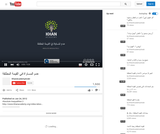Conditions of Use:
Remix and Share
Rating

This 8-minute video lesson continues to look at absolute inequalities.

Subject:
Algebra
Material Type:
Lecture
Provider:
Provider Set:
Author:
Khan, Salman
Date created
25-ذو القعدة-1433Conditions of Use:
Remix and Share
Rating

This 6-minute video lesson looks at absolute value and number lines.

Subject:
Algebra
Material Type:
Lecture
Provider:
Provider Set:
Author:
Khan, Salman
Date created
25-ذو القعدة-1433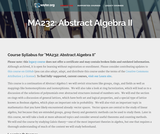Conditions of Use:
Read the Fine Print
Rating

This course is a continuation of Abstract Algebra I: the student will ...

This course is a continuation of Abstract Algebra I: the student will revisit structures like groups, rings, and fields as well as mappings like homomorphisms and isomorphisms. The student will also take a look at ring factorization, general lattices, and vector spaces. Later this course presents more advanced topics, such as Galois theory - one of the most important theories in algebra, but one that requires a thorough understanding of much of the content we will study beforehand. Upon successful completion of this course, students will be able to: Compute the sizes of finite groups when certain properties are known about those groups; Identify and manipulate solvable and nilpotent groups; Determine whether a polynomial ring is divisible or not and divide the polynomial (if it is divisible); Determine the basis of a vector space, change bases, and manipulate linear transformations; Define and use the Fundamental Theorem of Invertible Matrices; Use Galois theory to find general solutions of a polynomial over a field. (Mathematics 232)

Subject:
Algebra
Material Type:
Full Course
Provider:
The Saylor Foundation
Date created
15-ذو الحجة-1432Conditions of Use:
Remix and Share
Rating

This problem involves solving a system of algebraic equations from a context: ...

This problem involves solving a system of algebraic equations from a context: depending how the problem is interpreted, there may be one equation or two.

Subject:
Mathematics
Algebra
Material Type:
Activity/Lab
Provider:
Illustrative Mathematics
Provider Set:
Illustrative Mathematics
Author:
Illustrative Mathematics
Date created
1-جمادي الأولى-1434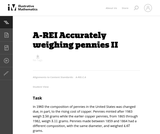Conditions of Use:
Remix and Share
Rating

This task is a somewhat more complicated version of "Accurately weighing pennies ...

This task is a somewhat more complicated version of "Accurately weighing pennies I'' as a third equation is needed in order to solve part (a) explicitly. Instead, students have to combine the algebraic techniques with some additional problem-solving (numerical reasoning, informed guess-and-check, etc.)

Subject:
Mathematics
Algebra
Material Type:
Activity/Lab
Provider:
Illustrative Mathematics
Provider Set:
Illustrative Mathematics
Author:
Illustrative Mathematics
Date created
1-جمادي الأولى-1434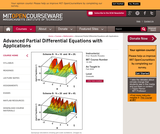Conditions of Use:
Remix and Share
Rating

" The focus of the course is the concepts and techniques for ...

" The focus of the course is the concepts and techniques for solving the partial differential equations (PDE) that permeate various scientific disciplines. The emphasis is on nonlinear PDE. Applications include problems from fluid dynamics, electrical and mechanical engineering, materials science, quantum mechanics, etc."

Subject:
Algebra
Material Type:
Full Course
Textbook
Provider:
M.I.T.
Provider Set:
M.I.T. OpenCourseWare
Author:
Rosales, Rodolfo R.
Date created
13-ربيع الأول-1432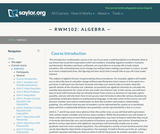Conditions of Use:
Read the Fine Print
Rating

This course discusses how to use algebra for a variety of everyday ...

This course discusses how to use algebra for a variety of everyday tasks, such as calculate change without specifying how much money is to be spent on a purchase, analyzing relationships by graphing, and describing real-world situations in business, accounting, and science.

Subject:
Algebra
Material Type:
Full Course
Provider:
The Saylor Foundation
Date created
21-شوال-1434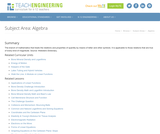Conditions of Use:
Read the Fine Print
Rating

The branch of mathematics that treats the relations and properties of quantity ...

The branch of mathematics that treats the relations and properties of quantity by means of letter and other symbols. It is applicable to those relations that are true of every kind of magnitude. Source: Websters Dictionary.

Subject:
Engineering
Algebra
Material Type:
Full Course
Provider:
TeachEngineering
Provider Set:
TeachEngineering
Author:
K-12 Outreach,
TeachEngineering.org
Date created
14-محرم-1436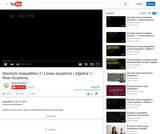Conditions of Use:
Remix and Share
Rating

This 8-minute video lesson continues to look at absolute inequalities.

Subject:
Algebra
Material Type:
Lecture
Provider:
Provider Set:
Author:
Khan, Salman
Date created
25-ذو القعدة-1433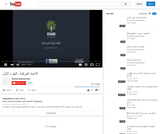Conditions of Use:
Remix and Share
Rating

An introduction to adding, subtracting and multiplying complex numbers. [Algebra: Lesson 34 ...

An introduction to adding, subtracting and multiplying complex numbers. [Algebra: Lesson 34 of 86]

Subject:
Algebra
Material Type:
Lecture
Provider:
Provider Set:
Author:
Khan, Salman
Date created
23-ذو القعدة-1433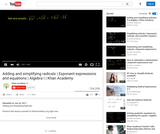Conditions of Use:
Remix and Share
Rating

This 5-minute video lesson looks at adding and simplifying radicals.

Subject:
Algebra
Material Type:
Lecture
Provider:
Provider Set:
Author:
Khan, Salman
Date created
25-ذو القعدة-1433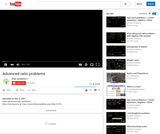Conditions of Use:
Remix and Share
Rating

A demonstration of the use of algebra for more advanced ratio problems.

Subject:
Algebra
Material Type:
Lecture
Provider:
Provider Set:
Author:
Khan, Salman
Date created
3-ربيع الأول-1433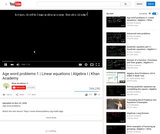Conditions of Use:
Remix and Share
Rating

This lesson introduces the use of algebra for solving age word problems.

Subject:
Algebra
Material Type:
Lecture
Provider:
Provider Set:
Author:
Khan, Salman
Date created
3-ربيع الأول-1433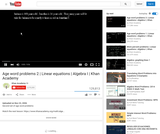Conditions of Use:
Remix and Share
Rating

This lesson continues the explanation for the use of algebra to solve ...

This lesson continues the explanation for the use of algebra to solve age word problems.

Subject:
Algebra
Material Type:
Lecture
Provider:
Provider Set:
Author:
Khan, Salman
Date created
3-ربيع الأول-1433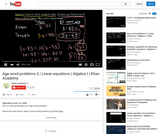Conditions of Use:
Remix and Share
Rating

A demonstration of using algebra to solve age word problems.

Subject:
Algebra
Material Type:
Lecture
Provider:
Provider Set:
Author:
Khan, Salman
Date created
3-ربيع الأول-1433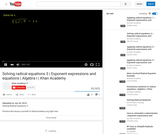Conditions of Use:
Remix and Share
Rating

This 4-minute video lesson continues to demonstrate how to solve radical equations.

Subject:
Algebra
Material Type:
Lecture
Provider: